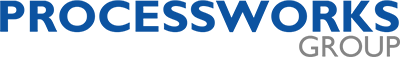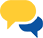Course Category: Software Process Engineering
Course Duration: 2 Days
Hours: 14 Contact Hours

This two-day course is essential for those who want to understand how software estimation can be used as a means of improving the prediction of ‘size’ in any application software. The course commences with a discussion on the need of Estimation and the key process parameters that are to be estimated in a project.

A number of alternative techniques for ‘size’ estimation (FPA, Quick FP, Use Case Point, WBS (Work Breakdown Structure) based) are briefly discussed, together with how these techniques could be applied in a given project context.

The course then moves on to the topics of other estimation parameters (effort, schedule). Participants will learn how to derive the effort and schedule estimates from the computed ‘size’ of the application software.

The theory presented during the course is supported by a number of practical exercises and case study that demonstrate the software estimation process.

## Course Objectives

•  A clear understanding of the role of estimation and how this relates to the goal of overall project execution.
• Learning the techniques for estimating ‘size’ of application software.
• Ability to relate with the different ‘size’ estimation techniques and understand limitations of the same.
• Understanding how to derive effort and schedule estimates based on ‘size’

## Course Outline

The two day course would cover the topics as noted below. There would be exercises at appropriate junction points to elaborate and understand the concepts.

### Estimation Concepts

• Some popular assumptions about estimation
• Risks in Estimation
• Cone of Uncertainty
• What to Estimate

### Process of Estimation

• Sequence of Estimation parameters
• Use of Historical Data
• Process Steps
• Size Measurement Methods and Estimation Models

### Function Point Counting

• The FPA Method
• Historical Perspective
• FP Counting Process
• Process Steps and Rules
• FPA Steps for Files
• FPA Steps for Transaction
• Count Unadjusted Data Function Point
• Count Unadjusted Transaction Function Point
• Final Function Point Count

### Quick Function Point Counting

• Need for Quick FP Counting
• Process Steps and Rules
• Computing Quick Function Point

### Use Case Point Counting

• Use Case Modelling concepts
• UML Use Case Diagrams
• System Described
• Actors interacting with System
• Use Cases
• Relationship of above elements
• Use Case Points
• Technical Complexity Factor
• Environmental Complexity Factor
• Productivity Factor

### WBS (Work Breakdown Structure) Based Estimation

• Need for FP Estimating (not Counting)
• Derived Estimation Method
• Sequence of operations
• What is WBS
• How to build WBS
• Relationship between project activities and work Item
• Use of Productivity parameter in WBS based Estimates
• Advantages of WBS Based Estimates
• Limitations of WBS Based Estimates

### Constructive Cost Model (COCOMO II)

• Understand Structure of COCOMO II
• The Basic Equation
• Economies and Diseconomies of Scale
• Compute Effort
• Calculate Schedule

### Delphi Wide Band Technique

• Relation of Delphi with WBS
• Delphi process
• Pointers for success in Delphi

Course Category: Software Process Engineering
Course Duration: 2 Days
Hours: 14 Contact Hours

Processworks Sdn Bhd
MyCOID: 659359-X
HRD Corp registered training providerEnquiries?